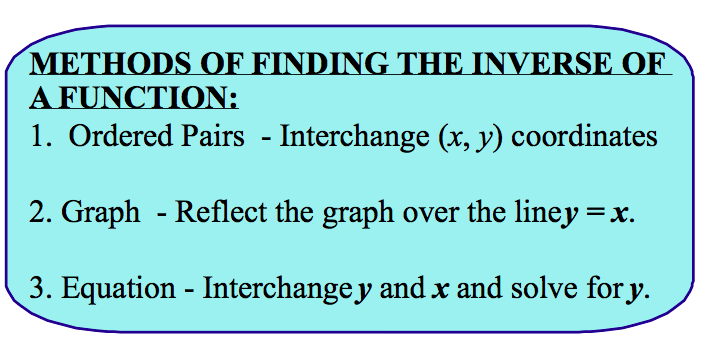# Unit 3!

` In this unit we will be discussing inverse functions, graphing square root & cube root functions, or solving radical equations.`

### Step 1: Write the equation

Question: The variable z varies jointly with x and y. Write and equation that relates x, y, and z. Then find z when x = -2 and y = 5. x = -2, y = 6, z = 18.

18 = a(-2)(6)

18 = a(-12)

### Step 3: Divide both sides of the equation by our new number

We do this so we can get a by itself

a = 18/-12 (simplified) = a = -3/2 or -1.5

### Step 4: Find z when a = -1.5, x = -2, y = 5

z = (-1.5)(-2)(5) = -1.5 x -2 = 3. 3 x 5 = 15. z = 15.